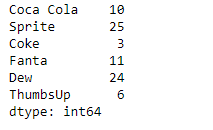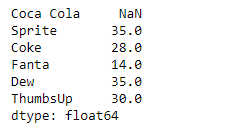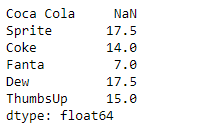Open in App
Not now

# Python | Pandas Series.rolling()

• Last Updated : 07 Feb, 2019

Pandas series is a One-dimensional ndarray with axis labels. The labels need not be unique but must be a hashable type. The object supports both integer and label-based indexing and provides a host of methods for performing operations involving the index.

Pandas` Series.rolling()` function is a very useful function. It Provides rolling window calculations over the underlying data in the given Series object.

Syntax: Series.rolling(window, min_periods=None, center=False, win_type=None, on=None, axis=0, closed=None)

Parameter :
window : Size of the moving window
min_periods : Minimum number of observations in window required to have a value
center : Set the labels at the center of the window.
win_type : Provide a window type.
on : str, optional
axis : int or str, default 0
closed : Make the interval closed on the ‘right’, ‘left’, ‘both’ or ‘neither’ endpoints.

Returns : a Window or Rolling sub-classed for the particular operation

Example #1: Use `Series.rolling()` function to find the rolling window sum of the underlying data for the given Series object. The size of the rolling window should be 2 and the weightage of each element should be same.

 `# importing pandas as pd``import` `pandas as pd`` ` `# Creating the Series``sr ``=` `pd.Series([``10``, ``25``, ``3``, ``11``, ``24``, ``6``])`` ` `# Create the Index``index_ ``=` `[``'Coca Cola'``, ``'Sprite'``, ``'Coke'``, ``'Fanta'``, ``'Dew'``, ``'ThumbsUp'``]`` ` `# set the index``sr.index ``=` `index_`` ` `# Print the series``print``(sr)`

Output :Now we will use `Series.rolling()` function to find the sum of the underlying data having a window size of 2.

 `# Find sum over a window size of 2``result ``=` `sr.rolling(``2``).``sum``()`` ` `# Print the returned Series object``print``(result)`

Output :As we can see in the output, the `Series.rolling()` function has successfully returned a series object having found the sum of the underlying data over a window size of 2. Notice the first value is a missing value as there was no element previous to it so the sum could not be performed.

Example #2: Use `Series.rolling()` function to find the rolling window sum of the underlying data for the given Series object. The size of the rolling window should be 2 and the rolling window type should be ‘triang’.

 `# importing pandas as pd``import` `pandas as pd`` ` `# Creating the Series``sr ``=` `pd.Series([``10``, ``25``, ``3``, ``11``, ``24``, ``6``])`` ` `# Create the Index``index_ ``=` `[``'Coca Cola'``, ``'Sprite'``, ``'Coke'``, ``'Fanta'``, ``'Dew'``, ``'ThumbsUp'``]`` ` `# set the index``sr.index ``=` `index_`` ` `# Print the series``print``(sr)`

Output :Now we will use `Series.rolling()` function to find the sum of the underlying data having a window size of 2.

 `# Find sum over a window size of 2``# We have also provided the window type``result ``=` `sr.rolling(``2``, win_type ``=``'triang'``).``sum``()`` ` `# Print the returned Series object``print``(result)`

Output :As we can see in the output, the `Series.rolling()` function has successfully returned a series object having found the sum of the underlying data over a window size of 2. Notice the first value is a missing value as there was no element previous to it so the sum could not be performed.

My Personal Notes arrow_drop_up